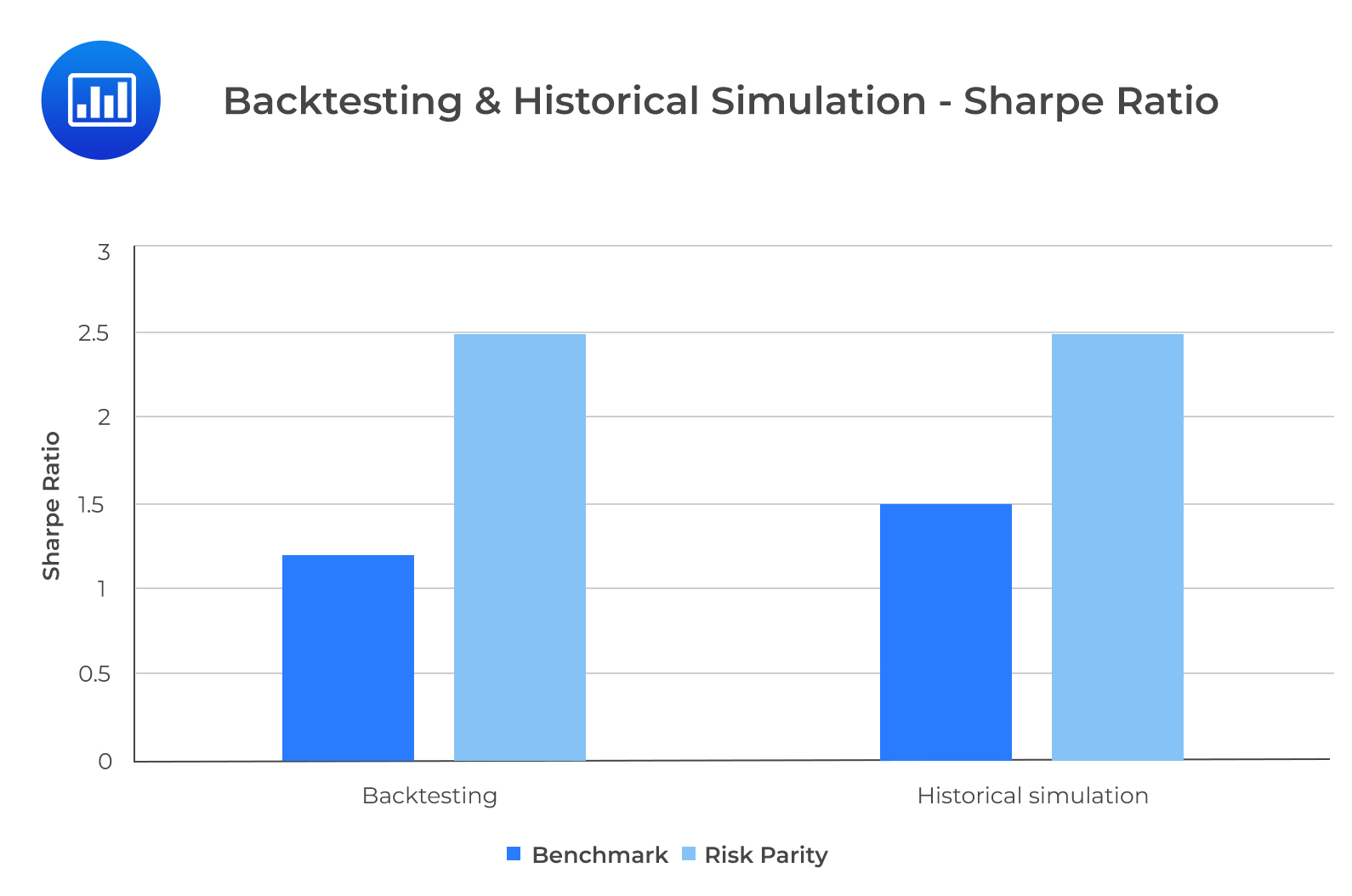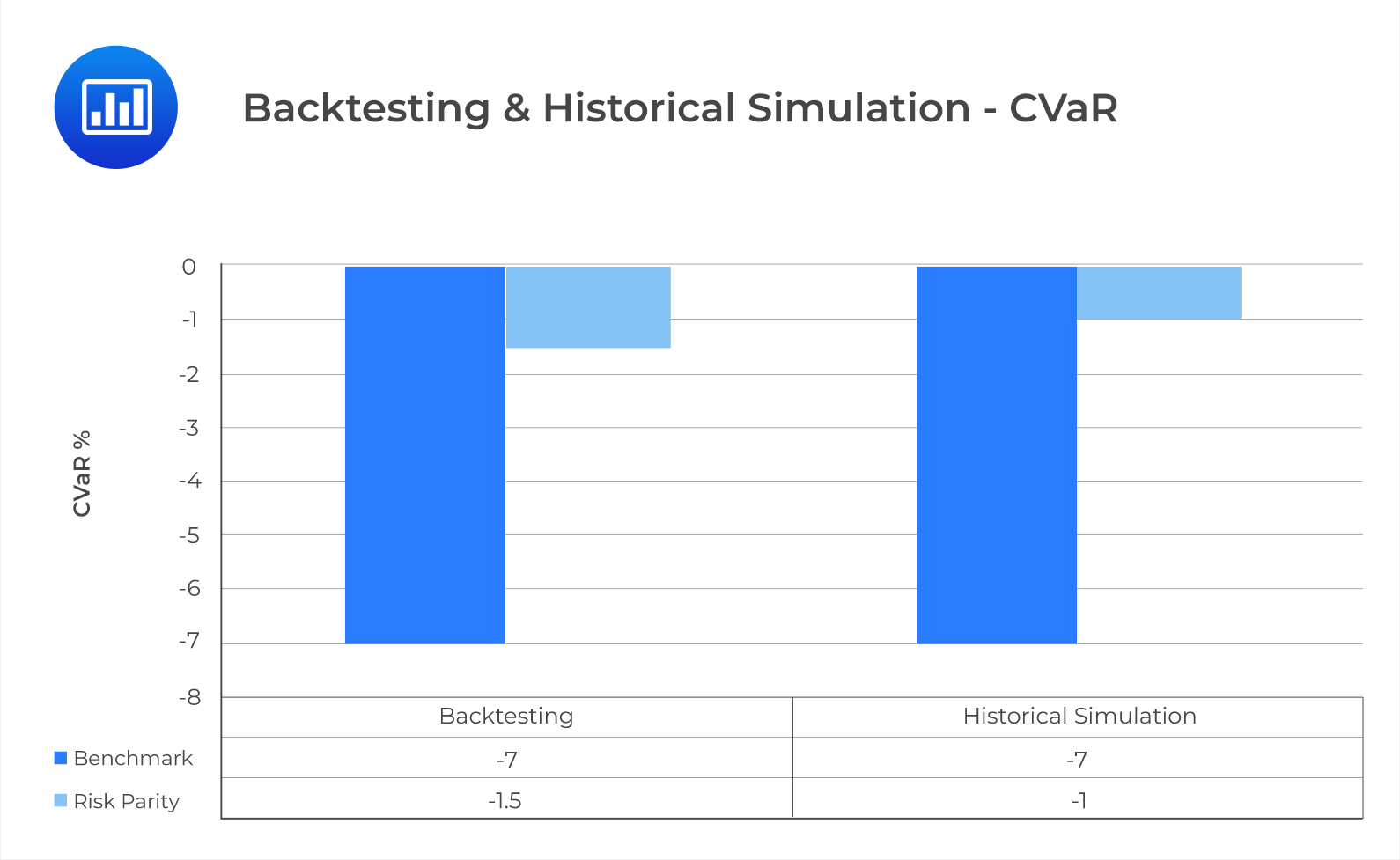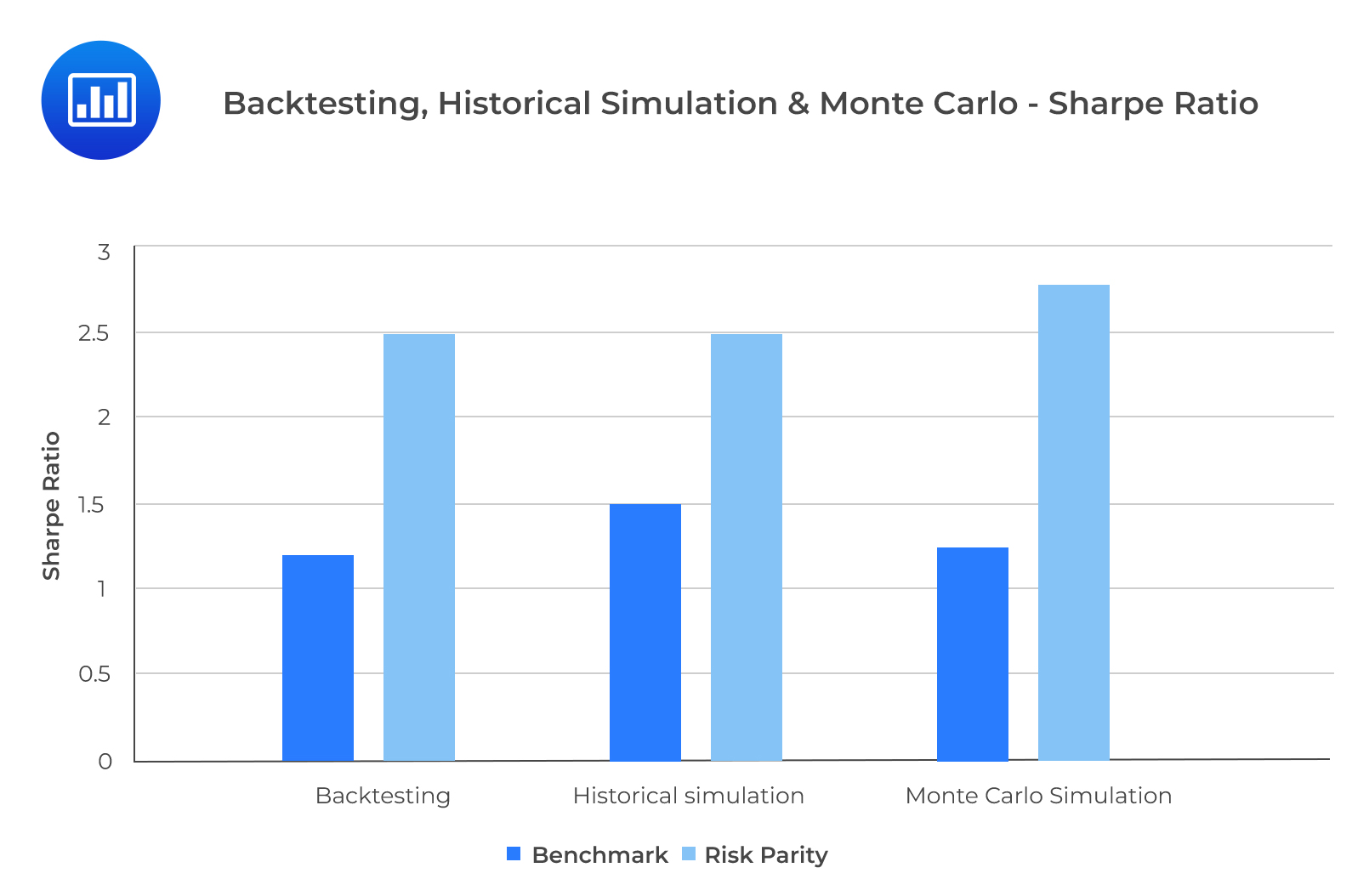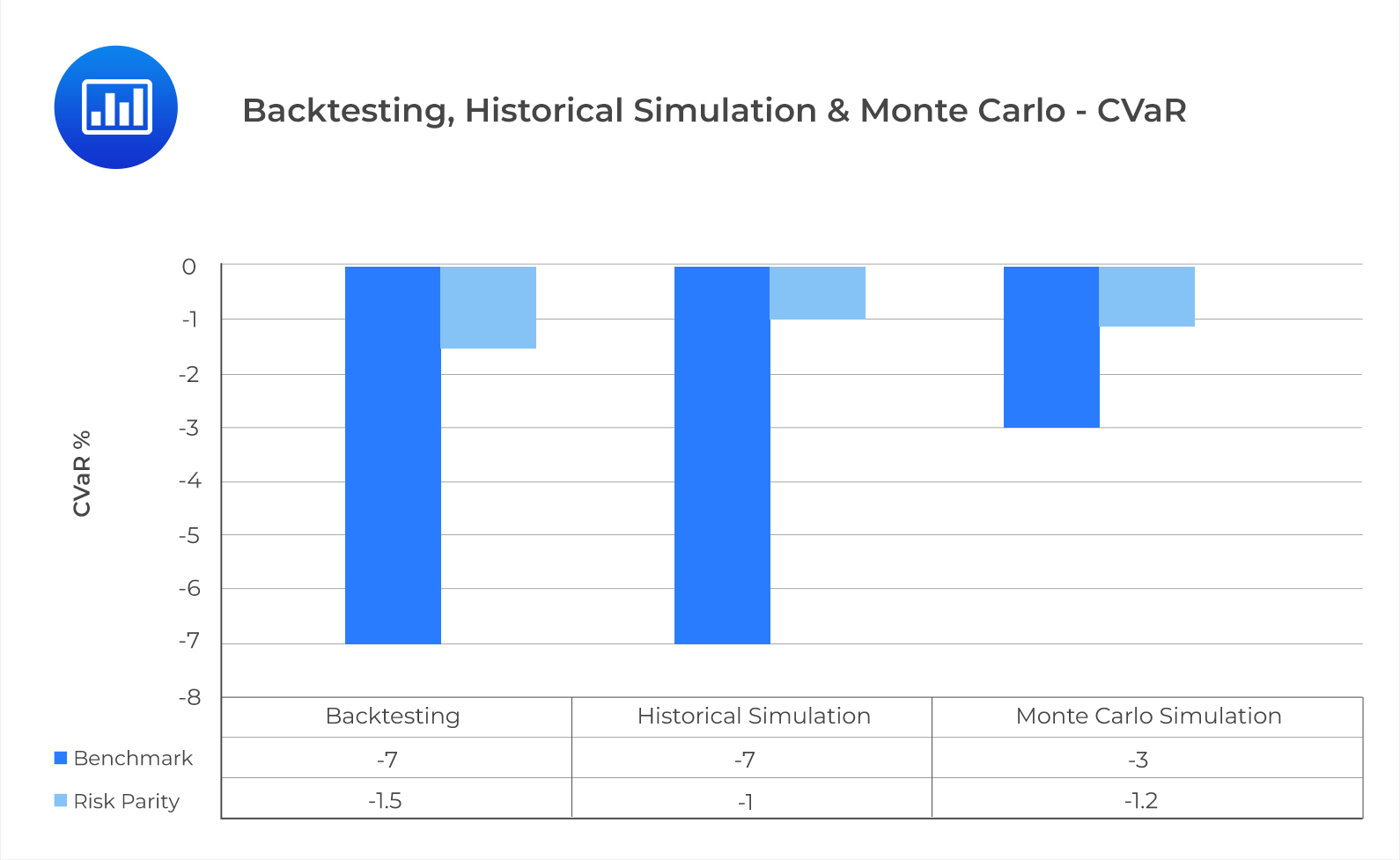Limited Time Offer: Save 10% on all 2022 Premium Study Packages with promo code: BLOG10# Inputs and Decisions in Simulation

## Historical Simulation

Historical simulation employs randomness by drawing returns from historical data randomly, instead of following each period chronologically. An election can be made on whether to sample from the historical returns with or without replacement. Analysts prefer drawing samples from historical returns without replacement since the number of simulations is larger, in this case, than the historical data size. Note that random sampling with replacement is also known as bootstrapping.

Let us use the factor allocation strategies for eight-factor portfolios to illustrate and interpret a simulation.

1. The target variables are the returns of the risk parity and benchmark multifactor portfolios.
2. The key decision variables are returns of the eight-underlying factor-based portfolios.
3. N = 800 trials.
4. Bootstrapping sampling will be used to implement the historical simulation. A random number will be drawn from the uniform distribution and assigned to a specific historical month between April 1988 and May 2019 (374 months). Numbers are assigned by dividing the span of the uniform distribution by the number of months $$(\frac{1}{374} = 0.00267)$$. We select random numbers that fall between 0 and 0.00267.
5. 800 numbers will be drawn randomly from a uniform distribution between 0 and 1 by the random number generator. This can be seen in Exhibit 4.
6. One month is selected at random. From the returns of the corresponding eight-factor portfolios, one possible outcome is selected and used to compute the values of the returns of the risk parity and benchmark portfolio. The return of the benchmark portfolio for October 2006 is $$(0.125\times2.5\%+0.125\times0.3\%+0.125\times-0.8\%+0.125\times0\%\\+0.125\times-0.75\%+0.125\times2.5\%+0.125\times0.5\%+0.125\times-0.6\%)\\= 0.456\%$$ and the return for one month of the risk parity portfolio is $$( 6.0\%\times2.5\%+30.3\%\times0.3\%+11.7\%\times-0.8\%+5.2\%\times0\%\\+10.4\%\times-0.75\%+6.3\%\times2.5\%+9.6\%\times0.5\%+20.4\%\times-0.6\%)\\ = 0.1524\%.$$
7. We repeat the same simulation process for steps 5 to 6 for the 800 trials. This will generate a collection of 800 simulated returns for the risk parity and benchmark portfolio.
8. Performance metrics can be calculated using the 800 scenarios.

### Exhibit 4: Factor Returns for Five Randomly Selected Months

$$\small{\begin{array}{l|c|c|c|c|c|c|c}\textbf{Simulation} & \textbf{Month} & {\textbf{Random}\\ \textbf{no}} & {\textbf{Month}\\ \textbf{no}} & {\textbf{Earnings}\\ \textbf{Yield}} & {\textbf{Book-to-}\\ \textbf{Market}} & {\textbf{Earnings}\\ \text{Growth}} & \textbf{Momentum} \\ \hline1 & 31/10/2006 & 0.595441 & 223 & 2.5\% & 0.3\% & -0.8\% & 0\% \\ \hline 2 & 31/5/1998 & 0.32574 & 122 & 0.1\% & 0.8\% & -0.2\% & -0.5\% \\ \hline 3 & 31/3/2012 & 0.76896 & 288 & -2\% & 0.6\% & 1.7\% & 1.8\% \\ \hline4 & 31/3/2016 & 0.89712 & 336 & 2.6\% & 2.5\% & -0.4\% & -1.5\% \\ 5 & 30/6/2002 & 0.45657 & 171 & 6.5\% & -3.4\% & 1.8\% & 2.4\%\\ \end{array}}$$

$$\small{\begin{array}{l|c|c|c|c|c|c|c} \textbf{Simulation} & \textbf{Month} & {\textbf{Random}\\ \textbf{no}} & {\textbf{Month}\\ \textbf{no}} & {\textbf{Earnings}\\ \textbf{Revision}} & \textbf{ROE} & \textbf{Debt/Equity} & {\textbf{Earnings}\\ \textbf{Quality}} \\ \hline 1 & 31/10/2006 & 0.595441 & 223 & -0.75\% & 2.5\% & 0.5\% & -0.6\% \\ \hline 2 & 31/5/1998 & 0.32574 & 122 & -0.1\% & -0.1\% & 0.3\% & 1.6\% \\ \hline 3 & 31/3/2012 & 0.76896 & 288 & 2\% & -0.6\% & -2.02\% & -0.9\% \\ \hline 4 & 31/3/2016 & 0.89712 & 336 & -1.6\% & 1.5\% & -1.2\% & 1.4\% \\ \hline 5 & 30/6/2002 & 0.45657 & 171 & 2.5\% & 6.4\% & -0.8\% & 1.3\%\\ \end{array}}$$

From Exhibit 5, the Sharpe ratios of the RP and BM strategies are in line with those of the rolling window backtesting. In both approaches, the BM portfolio is outperformed by the RP portfolio according to the Sharpe ratio. For both approaches, RP carries less substantial downside risk than the BM portfolio.

### Exhibit 5: Comparing Backtesting With Historical Simulation## Monte Carlo Simulation

One problem with historical simulation is that it uses past data that may not represent the future. To address this problem, we will use the Monte Carlo simulation. The steps for both methodologies are similar, with a few key differences which we will highlight in this section. First, we have to specify a functional form for each key decision variable. Exploratory data analysis is often important here. The usefulness of Monte Carlo simulation depends on how well the functional forms reflect the true distribution of the underlying data.

A few key considerations need to be made before we can finalize our choice of functional forms.

• The distribution should describe the key empirical patterns of the underlying data.
• Correlations between multiple key decision variables need to be properly accounted for.
• The complexity of the functional form and the number of parameters that determine the functional form are important.

Using the RP and BM strategies, the Monte Carlo simulation can be carried out as follows:

1. The returns for the BM and RP multifactor portfolios will be our target variables.
2. The returns of the eight-underlying factor-based portfolios are our key decision variables.
3. 800 trials will be performed for this simulation.
4. The initial functional form is the multivariate normal distribution. We will calculate the eight standard deviations, the eight-factor portfolio mean returns and the 28 elements of the covariance matrix of historical factor return data from April 1988 to May 2019.
5. We use the calibrated multivariate normal distribution to simulate the future factor returns. Exhibit 6 illustrates this.
6. We compute the value of the target variables once the returns of the eight-factor portfolio have been simulated. For simulation 1, the benchmark portfolio delivers a monthly return of $$(0.125\times-3\%+0.125\times-3.2\%+0.125\times0.7\%+0.125\times2.4\%+\\0.125\times-3.3\%+0.125\times-0.3\%+0.125\times-1.8\%+0.125\times2\%)\\ = -1.312\%$$ and the return for one month of the risk parity portfolio is $$(6.0\%\times-3\%+30.3\%\times-3.2\%+11.7\%\times-0.3\%+5.2\%\times0.7\%+\\10.4\%\times2.4\%+6.3\%\times-3.3\%+9.6\%\times-1.8\%+20.4\%\times-2\%) \\= – 1.69\%.$$ Repeat Steps 5 and 6 for 800 trials until we generate a collection of 800 returns for the risk parity and the benchmark portfolios.
7. We assess the risk profiles and performance of our two investment strategies from the 800 simulated returns.

### Exhibit 6:The First Five Simulations of Factor Return Using a Multivariate Normal Distribution

$$\small{\begin{array}{l|c|c|c|c|c|c|c|c}{\textbf{Simulation}\\ \textbf{no}} & {\textbf{Earnings}\\ \textbf{Yield}} & {\textbf{Book-to-}\\ \textbf{Market}} & {\textbf{Earnings}\\ \textbf{Growth}} & \textbf{Momentum} & {\textbf{Earnings}\\ \textbf{Revision}} & \textbf{ROE} & \textbf{Debt/Equity} & {\textbf{Earnings}\\ \textbf{Quality}} \\ \hline 1 & -3\% & -3.2\% & -0.3\% & 0.7\% & 2.4\% & -3.3\% & -1.8\% & 2\% \\ \hline 2 & 0\% & 3.7\% & 1\% & -0.5\% & 1\% & -2.3\% & -3.5\% & -0.2\% \\ \hline 3 & 0.8\% & -2\% & 3\% & 3.9\% & 2.6\% & 1.3\% & -0.9\% & 0\% \\ \hline 4 & 9.5\% & -0.4\% & 1.2\% & 3.8\% & -1\% & 7.7\% & -3.8\% & 1.7\% \\ \hline 5 & 1.8\% & 0.3\% & 2.9\% & -0.3\% & 3.2\% & 0.3\% & -1\% & 0.3\%\\ \end{array}}$$

### Exhibit 7: Comparing Backtesting, Historical Simulation, and Monte Carlo SimulationFrom exhibit 7, we can see that the RP strategy outperformed the BM strategy according to the Sharpe ratio in all three approaches. The CVar is slightly more sensitive to randomness. Compared to rolling window backtesting and historical simulation, it is under the Monte Carlo simulation that the downside risk is most understated for the BM strategy. This is because the factor returns for the Monte Carlo simulation are negatively skewed with excess kurtosis. The underestimation of risk only appears for the BM strategy because the correlations and the factor risks are not properly accounted for in the equal weighing scheme.

## Question

What could be the most likely reason why the downside risk of the benchmark strategy would be underestimated under the Monte Carlo simulation:

1. BM strategy is robust to non-normal factor distribution.
2. The correlations and the factor risks are properly accounted for in the equal weighing scheme.
3. The correlations and the factor risks are not properly accounted for in the equal weighing scheme.

#### Solution

The correct answer is C.

The underestimation of risk only manifests in the BM strategy because the correlations and the factor risks are not properly accounted for in the equal weighing scheme.

A is incorrect. The risk parity strategy is robust to a non-normal factor return distribution.

B is incorrect. The underestimation of downside risk would not occur if the correlations and the factor risks were accurately accounted for.

Reading 41: Backtesting and Simulation

LOS g): Explain inputs and decisions in simulation and interpret a simulation

Shop CFA® Exam Prep

Offered by AnalystPrepLevel I
Level II
Level III
All Three Levels
Featured Shop FRM® Exam PrepFRM Part I
FRM Part II
FRM Part I & Part II
Learn with Us

Subscribe to our newsletter and keep up with the latest and greatest tips for success
Shop Actuarial Exams PrepExam P (Probability)
Exam FM (Financial Mathematics)
Exams P & FM
Shop GMAT® Exam PrepComplete CourseDaniel Glyn
2021-03-24
I have finished my FRM1 thanks to AnalystPrep. And now using AnalystPrep for my FRM2 preparation. Professor Forjan is brilliant. He gives such good explanations and analogies. And more than anything makes learning fun. A big thank you to Analystprep and Professor Forjan. 5 stars all the way!michael walshe
2021-03-18
Professor James' videos are excellent for understanding the underlying theories behind financial engineering / financial analysis. The AnalystPrep videos were better than any of the others that I searched through on YouTube for providing a clear explanation of some concepts, such as Portfolio theory, CAPM, and Arbitrage Pricing theory. Watching these cleared up many of the unclarities I had in my head. Highly recommended.Nyka Smith
2021-02-18
Every concept is very well explained by Nilay Arun. kudos to you man!2021-02-13